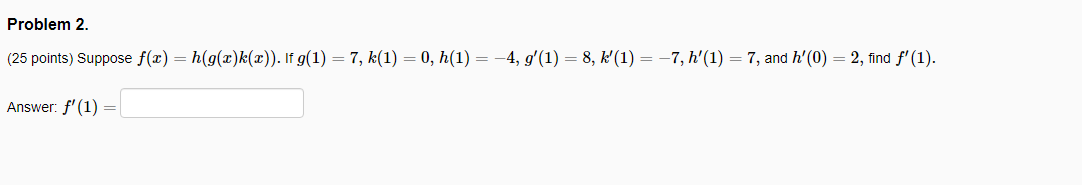### Create an Account

Home / Questions / Problem 2. (25 points) Suppose f(2)=h(g(2)k(2)). If g(1) = 7, k(1) = 0, h(1) = -4, g'(1) =...

# Problem 2. (25 points) Suppose f(2)=h(g(2)k(2)). If g(1) = 7, k(1) = 0, h(1) = -4, g'(1) = 8, K'(1) = -7, h'(1) = 7, and h'(0) = 2, find f'(1). Answer: f'(1) =

Problem 2. (25 points) Suppose f(2)=h(g(2)k(2)). If g(1) = 7, k(1) = 0, h(1) = -4, g'(1) = 8, K'(1) = -7, h'(1) = 7, and h'(0) = 2, find f'(1). Answer: f'(1) =Apr 25 2021 View more View LessSubscribe To Get Solution